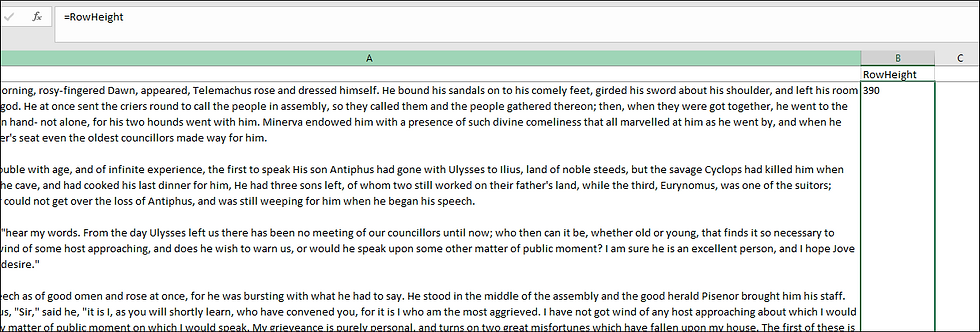top of pageSearch

# Reformatting Rows With Cut-Off Text

It very common after writing an Excel spreadsheet to a PDF or printing it out as a hard copy, to find that text in a cell has been cut-off. A cell can hold more text than it can display. The maximum height of a cell is 409.5. It's always possible to increase the amount of displayed text by increasing the width of the column (at least to a max of 255 characters), but a wider column may still not be able to show all of the text on landscaped letter or legal paper.

Here's how to deal with this problem. Note that his solution may need to be adjusted based on the number of returns used in a cell, and whether there is so much text that it must be divided into three or more rows.

1. The Tip of the Night for October 10, 2018, showed how to calculate the height of each row on a worksheet. List the heights in a column to the right of the column holding more text than can be displayed.2. Next enter the macro posted here,

Function FindN(sFindWhat As String, _

sInputString As String, N As Integer) As Integer

Dim J As Integer

Application.Volatile

FindN = 0

For J = 1 To N

FindN = InStr(FindN + 1, sInputString, sFindWhat)

If FindN = 0 Then Exit For

Next

End Function

. . . in new module in Visual Basic. This will allow you to enter a new formula:

=findN(CHAR(10),A2,3). . . to find the 3rd occurrence of a return (denoted by CHAR(10)) in cell A2.

This will find the Nth occurrence of returns (denoted by CHAR(10)) in the cell holding the lengthy text. In this example we're finding how many characters into a cell the 3rd occurrence of a return occurs. If you wanted to find the 4th occurrence, we'd change the end of the formula to, "+4)".

3. Next use the LEFT formula to pull the text from the beginning of cell A2 until the 3rd occurrence of a return character: =LEFT(A2,C2)We have put the first half of the text cell into a new cell.

4. Next minus the 3rd occurrence of the return character form the total character count of cell A2. We use the LEN formula to find the total character count.

=LEN(A2)-C2

5. In the next column to the right, enter the RIGHT formula making reference to the cell holding the value of the total character count minus the 3rd occurrence of the return character.6. Going back to the column containing the row height, filter it for only the rows at the 409.5 maximum. (It will be necessary to have a control number in one column so you can sort the spreadsheet into its original order.) Copy both the control number and the result from the LEFT formula into the first two columns at the end of the spreadsheet, then do the same with the result from the RIGHT formula. Shade the two extracts different colors.7. Sort the spreadsheet by the control number, and then also sort by the color of the original text, the first half extract and the second half extract8. We end up with the text successfully split into two cells.bottom of page